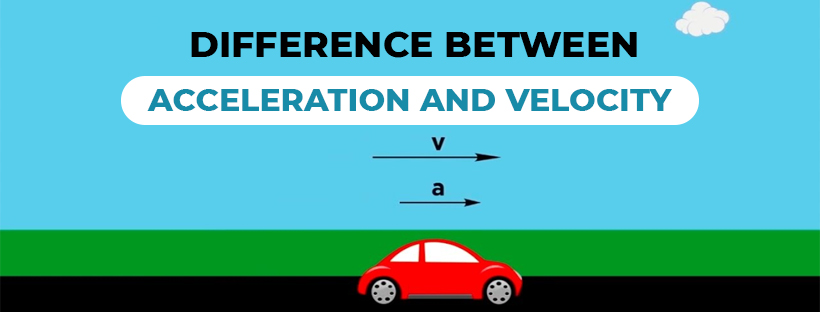Difference between Acceleration and Velocity - Study24x7Default error msg

New to Study24x7 ?

# Difference between Acceleration and Velocity

Updated on 21 January 2021Class12NonMedical
Updated on 21 January 2021The difference between acceleration and velocity is one of the most asked questions in physics board exams as well as in many top entrance exams like JEE, NEET, etc. We will also look at the difference between speed and velocity, acceleration and retardation, deceleration, etc further in this article to know the terms better. But before proceeding, it is important to know their definitions or what does this term denotes.

Both acceleration and velocity are the two major terms associated with the motion of objects. In simpler words-

Velocity is the rate of displacement of a moving object over time

Acceleration is defined as the rate of change of velocity over time.

Example- If you are walking at a constant speed, you have velocity but no acceleration and if your speed is changing while traveling, you have both velocity and acceleration.

Now, you have a gist of both the terms but understanding them completely is significant before differentiating between the two.

## What is Velocity?

Velocity is the rate of change of your position over a while. In layman's terms velocity is the same as speed however in physics there is a significant distinction among the two terms. Where "speed" is scalar quantity velocity is a "vector" quantity which means it has both speeds as well as directions.

Example- If we say X is traveling at 10 meters per second then this is a speed and if we say X is traveling at 10 meters per second in the south direction then this is the velocity because the latter consists of velocity too. From here we get the formulae to calculate velocity i.e.-Velocity= Distance Traveled/ Time Taken

## What is Acceleration?

Acceleration depends upon velocity as this means the rate of change in velocity within a period. When there is an increase in velocity it is known as acceleration however when there is a decrease in velocity it is termed as deceleration. Acceleration can be defined simply as-

Acceleration= Change in Velocity/ Time Taken for velocity to change.

With the following comparison chart between the two, you will be able to get the difference between acceleration and velocity more aptly.

 Basis of Comparison Velocity Acceleration Definition Velocity is associated with the speed of an object in the given direction. Acceleration is defined as the change in velocity of an object over a while Meaning Rate of change of position Rate of change of velocity Unit of Measurement Meter/second (m/s) Meter/second2 (m/s2) Determines How fast an object is moving including the direction How fast velocity changes with a period Calculated with Displacement Velocity Nature Vector Vector

## Difference between Speed and Velocity

 Differentiating property Speed Velocity Definition The rate at which an object covers a certain distance is known as speed The rate at which an object changes a position in a certain direction is known as acceleration Nature Scalar Quantity Vector Quantity Magnitude Speed can never be zero or negative Velocity can be zero, negative or positive Relation Speed may or may not be equal to the velocity Velocity can be different but speed remains the same Change in Direction The speed of the object did not change even with the change in direction The velocity of the object changes with change in direction

## Difference between Acceleration and Retardation

Retardation is known as a decrease of velocity per unit of time, it is just the opposite of acceleration. It generally means slowing down or losing speed. Acceleration can be positive as well as negative and this negative acceleration is known as retardation or deceleration. SI unit of retardation is just the opposite of acceleration, i.e. m/s^2

Example- The velocity of the car decreases when the brake is applied and produces negative acceleration thus known as retardation.

This was the whole difference between acceleration and retardation that students need to know and understand.

## Conclusion

Acceleration and velocity are both vector units hence they are connected with a magnitude as well as direction. Unlike speed, their values can be positive, negative, and zero according to the change.

We have seen the difference between acceleration and velocity above. So, now we can conclude by stating that velocity is not an outcome of acceleration but acceleration is surely an outcome of velocity because an object with acceleration certainly has variable velocity.

Related Article:

Difference between Distance and Displacement

Write a comment...Trending ArticlesBy CBSE CLASS 8By CBSE CLASS 10By Edubabble LLPBy CBSE CLASS 7
Related PostsServicesNeed Some Help!Connect